CFD-DEM耦合计算中的体积分数算法

Volume fraction allocation using characteristic points for coupled CFD-DEM calculations
LIU Detian, FU Xudong, WANG Guangqian
State Key Laboratory of Hydroscience and Engineering, Tsinghua University, Beijing 100084, China
Abstract: The coupled CFD-DEM method has been widely used for multiphase flows where the fluid volume fraction links the continuous medium (fluid) and the discrete medium (particles). This paper presents an improved volume fraction allocation algorithm based on the traditional SKM (statistical kernel method) model with subdividing of the characteristic points. Spatially distributed characteristic points within the region influenced by a particle mark all the CFD cells influenced by the particle so that the particle volume is correctly decomposed into each CFD cell. A traditional grid search algorithm then recognizes the characteristic points. After calibration of the model parameters, the algorithm reduces the truncation error at inner boundaries in parallel computing models. Numerical tests show that the algorithm is effective and efficient when the particle diameter size D and the CFD cell size L are of the same order of magnitude. The model reverts to the traditional unresolved particle model for LD and to the resolved particle model for DL. The algorithm improves coupled CFD-DEM calculations having a wide range of particle diameters to improve solid-liquid two-phase flow simulations.
Key words: CFD-DEM coupling     volume fraction     subdividing characteristic points     parallel calculation     computational efficiency

CFD-DEM耦合方法已越来越广泛地应用于多相流研究，如推移质输移、流化床反应等。在CFD-DEM耦合方法中，流体运动由Euler框架下的连续方法描述，固体颗粒在考虑粒间碰撞及颗粒-流体作用的基础上由Lagrange方法追踪。由于Lagrange框架下的离散方法仅用来描述系统的微观特性，在空间和时间尺度上远小于连续性方法所描述的宏观特性，因此需要准确、高效地建立宏观尺度(连续介质)与微观尺度(离散介质)间的桥梁，即流体体积分数场。

PCM是一种简单的体积分数场算法。它将颗粒的全部体积归属于颗粒中心所在的CFD网格，当颗粒中心临近CFD网格边缘时，引起的误差可能达到50%。同时，当一个CFD网格中包含多个颗粒时，全部颗粒体积累加可能大于CFD网格体积；当一个CFD网格中仅包含一个颗粒时，该颗粒体积也可能大于CFD网格体积。这种非物理的不光滑结果将导致整个CFD-DEM模拟计算的数值不稳定。因此，PCM常用于CFD网格尺寸显著大于颗粒尺寸(如L约为3~10倍D)的情形

DPVM最早由Wu等提出，用于解决当颗粒覆盖多个CFD网格时PCM所引起的较大误差。DPVM将颗粒体积按其实际位置在所覆盖的CFD网格中分配。相对于PCM，DPVM能得到相对光滑的体积分数场，模拟结果的准确性有显著提升。但是，由于颗粒体积在不同网格中的分配与网格形状、拓扑关系等有关，DPVM在非均匀网格中较难实现，也无法解决DL时非物理计算结果所引起的数值不稳定问题。

SKM将颗粒体积依据内核函数在整个计算域内进行加权分配，内核函数包含盒式分布函数(此时，SKM退化为PCM)、Gauss分布函数和Johnson's SB分布函数等。计算域内某一点的体积分数由不同颗粒在该点处的体积权重叠加得到。在DL时，仍能够得到光滑的体积分数场，保证耦合计算的数值稳定性。但由于颗粒体积在整个计算域内分配，需要对每个颗粒所影响的CFD网格进行循环搜索，计算代价高昂。当实施并行计算时，因独立计算的子区域之间要通过子区域交界面的信息交互实现多个处理器的协同并发计算，颗粒影响范围往往穿越不同处理器负责区域之间的内部边界，在内部边界处截断颗粒影响范围将导致颗粒体积不满足守恒条件、产生误差可达50%。这为SKM的广泛应用，特别是在大规模并行计算中的应用带来挑战[9-10]

1 改进型SKM算法

 ${h_i} = h\left( {x - {x_i}} \right) = \frac{1}{{{{\left( {{b^2}{\rm{\pi }}} \right)}^{3/2}}}}\exp \left[ { - \frac{{{{\left( {x - {x_i}} \right)}^{\rm{T}}}\left( {x - {x_i}} \right)}}{{{b^2}}}} \right].$ (1)

 $\int {h\left( x \right){\rm{d}}x} = 1.$ (2)

 $\alpha \left( x \right) = \sum\limits_{i = 1}^{{N_p}} {{V_{p,i}}{h_i}} = \sum\limits_{i = 1}^{{N_p}} {{V_{p,i}}h\left( {x - {x_i}} \right)} .$ (3)图 1 特征点剖分方法示意图

1) 确定颗粒影响范围。以颗粒i的球心(坐标xi)为中心、以搜索半径R为参数，确立颗粒空间影响范围(即搜索半径R覆盖区域的外接立方体)。

2) 布置特征点。在立方体内三维方向均匀布置特征点，每个方向上的特征点间距为该方向CFD网格的最小间距(以位于xy平面上的特征点为例，如图 1b中的LxLy)，若计算域在xy方向的长度分别为LXLY，则特征点在此平面上的坐标为

 $\begin{array}{*{20}{c}} {{x_c} = {x_{j,k}} = \left( {{x_j},{y_k}} \right) = \left( {\left( {\frac{1}{2} + j} \right){L_x},\left( {\frac{1}{2} + k} \right){L_y}} \right),}\\ {j = \left( {0,1,2, \cdots ,{\mathop{\rm int}} \left[ {{L_X}/{L_x}} \right] - 1} \right),}\\ {k = \left( {0,1,2, \cdots ,{\mathop{\rm int}} \left[ {{L_Y}/{L_y}} \right] - 1} \right).} \end{array}$ (4)

3) 确定有效特征点。特征点中心到颗粒中心距离小于搜索半径R时为有效特征点，反之为无效特征点，即有效特征点满足：

 $\left| {{x_i} - {x_c}} \right| < R.$ (5)

4) 标记CFD网格。通过搜索有效特征点所属的CFD网格，标记该颗粒所影响的所有CFD网格。因特征点间距为CFD网格间距最小值，因而所有被影响的CFD网格都会被标记。当不同特征点属于同一网格时，只标记一次，如图 1b所示，从而使颗粒体积守恒条件满足。以位于xy平面上的特征点为例，若当前网格中心坐标为xmi=(xmi, ymi)，且网格在xy方向上长度分别为LmxLmy，则被标记网格满足：

 $\begin{array}{l} \;\;\;\;\;\;\;\;\;\;\;\;\;\left| {\left( {\frac{1}{2} + j} \right){L_x} - {x_{mi}}} \right| < \frac{1}{2}{L_{mx}}\\ 且\;\;\;\;\;\;\;\;\left| {\left( {\frac{1}{2} + k} \right){L_y} - {y_{mi}}} \right| < \frac{1}{2}{L_{my}}. \end{array}$ (6)

5) 计算CFD网格体积权重。完成网格标记后，以颗粒中心坐标为xi，以CFD网格中心坐标为xmi，由式(1) 计算该颗粒在空间位置xmi处产生的体积权重值：

 $\begin{array}{*{20}{c}} {{h_{mi}} = h\left( {{x_{mi}} - {x_i}} \right) = }\\ {\frac{1}{{{{\left( {{b^2}{\rm{\pi }}} \right)}^{3/2}}}}\exp \left[ { - \frac{{{{\left( {{x_{mi}} - {x_i}} \right)}^{\rm{T}}}\left( {{x_{mi}} - {x_i}} \right)}}{{{b^2}}}} \right].} \end{array}$ (7)

6) 对所有颗粒进行循环。按照式(3) 将所有对空间位置x处有影响的颗粒体积权重值叠加，得到该时刻空间位置x处的体积分数值，实现从Lagrange场到Euler场的信息转化。

 ${T_1} = 1 + {6^1} + {6^2} + {6^3} + \cdots + {6^N} = \frac{1}{5}\left( {{6^{N + 1}} - 1} \right).$ (8)

 ${T_2} = {\left( {2N - 1} \right)^3}.$ (9)

 $\Delta T = M\left( {{T_1} - {T_2}} \right).$ (10)

M较大时，特征点剖分方法节省的计算耗时将是十分显著的。该方法提高了SKM的计算效率，也可以根据计算精度要求调整特征点分布数量。当特征点间距小于CFD网格最小尺寸时，可以保证识别全部CFD网格；当精度要求不高时，也可以减少特征点数量，以进一步提高计算效率。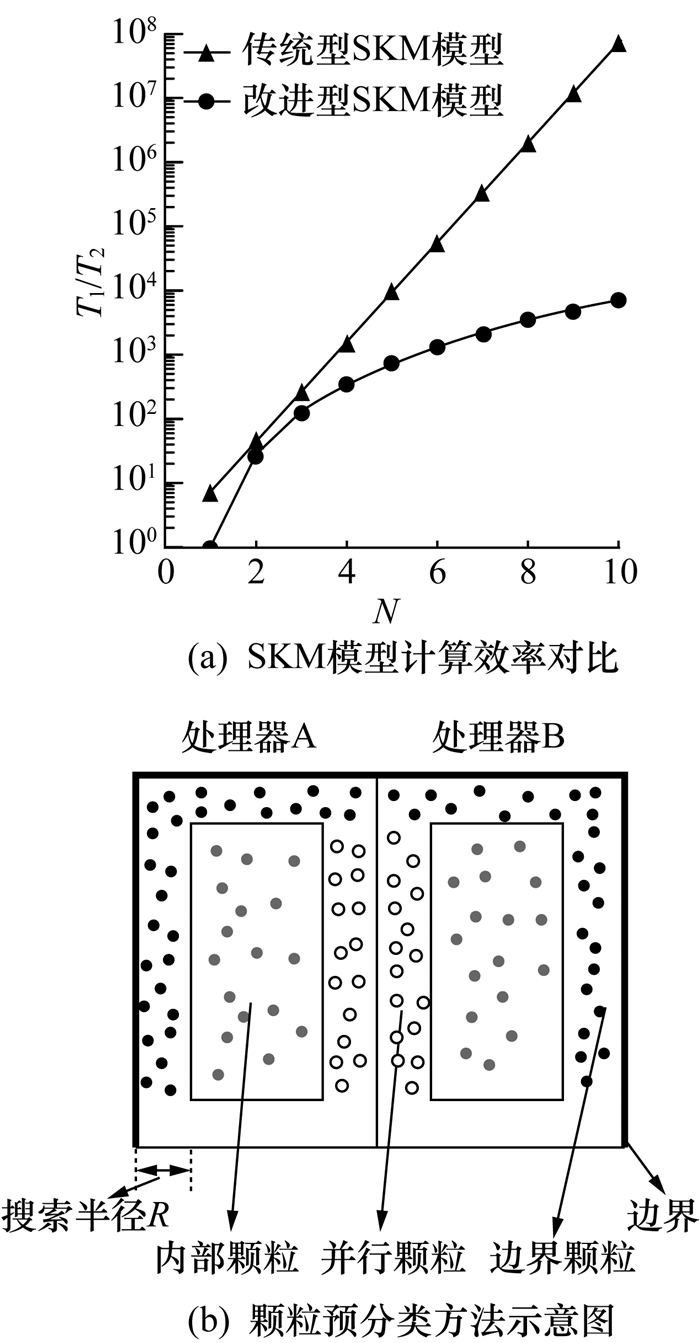图 2 特征点剖分方法的计算效率

2 静态颗粒排列实验

2.1 算例设置图 3 静态颗粒排列算例设置示意图

2.2 模型参数确定

 $\frac{b}{D} = 0.2615\frac{R}{b} + 0.3234.$ (11)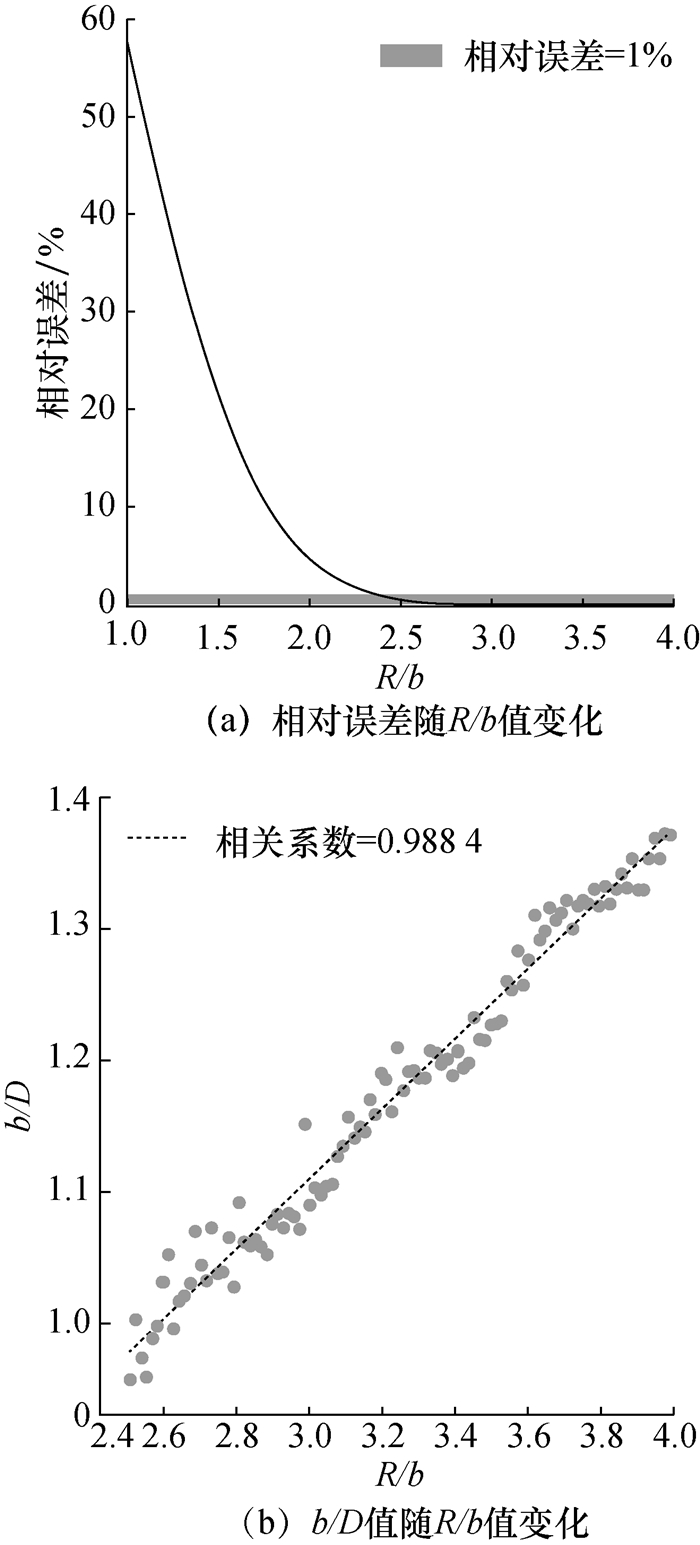图 4 改进型模型的参数确定

2.3 模型验证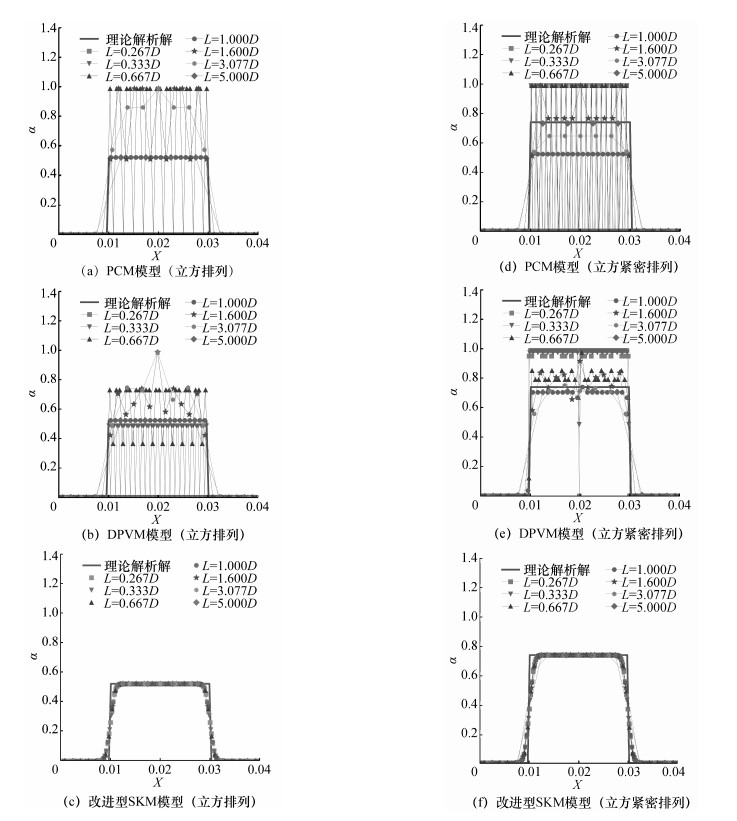图 5 均匀网格分布不同模型结果对比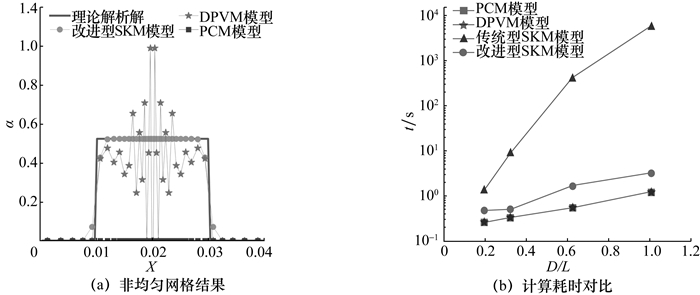图 6 不同模型非均匀网格及计算耗时结果

3 静水沉速实验检验

Concha提出采用如下公式计算静水中的颗粒沉速：

 ${u^ * } = \frac{{20.52}}{{{d^ * }}}{\left[ {{{\left( {1 + 0.0921{d^{ * 3/2}}} \right)}^{1/2}} - 1} \right]^2}.$ (12)

 ${d^ * } = \frac{D}{P},\;\;\;{u^ * } = \frac{u}{Q}.$ (13)

 $P = {\left( {\frac{3}{4}\frac{{\mu _{\rm{f}}^2}}{{\Delta \rho {\rho _{\rm{f}}}g}}} \right)^{1/3}},Q = {\left( {\frac{4}{3}\frac{{\Delta \rho {\mu _{\rm{f}}}g}}{{\rho _{\rm{f}}^2}}} \right)^{1/3}}.$ (14)

3.1 算例设置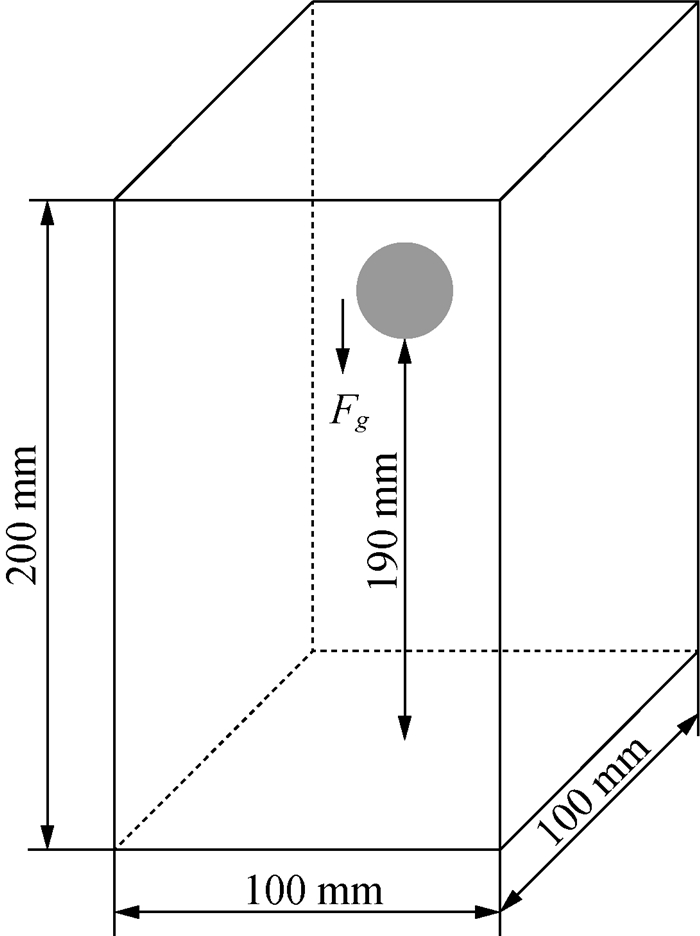图 7 静水沉速实验算例设置示意图

 ρf/(kg·m-3) μf/(N·s·m-2) L/D 算例1 970 0.373 0.3, 0.6, 1.5, 3, 4.5 算例2 965 0.212 0.3, 0.6, 1.5, 3, 4.5 算例3 962 0.113 0.3, 0.6, 1.5, 3, 4.5 算例4 960 0.058 0.3, 0.6, 1.5, 3, 4.5

3.2 计算结果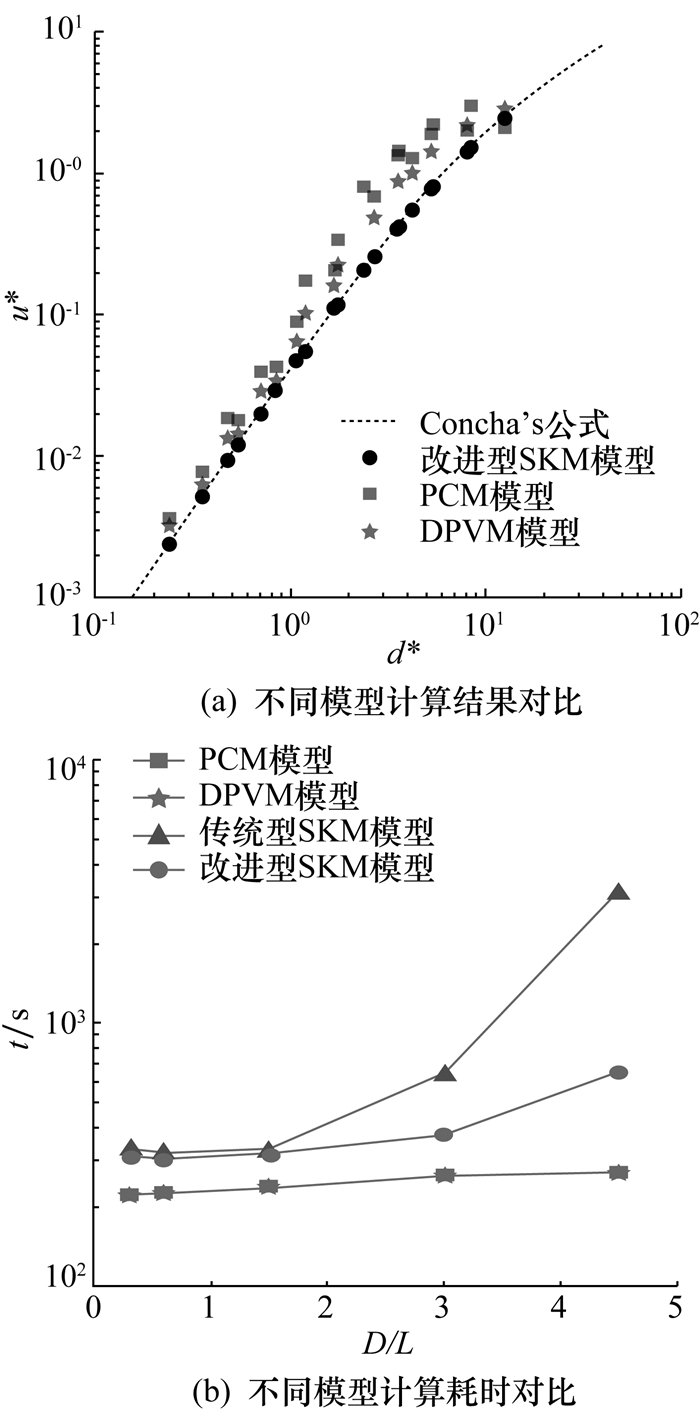图 8 静水沉速实验不同模型计算结果及计算耗时对比

4 结论

  Belytschko T, XIAO Shaoping, Schatz G C, et al. Atomistic simulations of nanotube fracture[J]. Physical Review B, 2002, 65: 2354301–2354308.  XIAO Shaoping, Belytschko T. A bridging domain method for coupling continua with molecular dynamics[J]. Computer Methods in Applied Mechanics & Engineering, 2004, 193(17-20): 1645–1669.  PENG Zhengbiao, Doroodchi E, LUO Caimao, et al. Influence of void fraction calculation on fidelity of CFD-DEM simulation of gas-solid bubbling fluidized beds[J]. AIChE Journal, 2014, 60(6): 2000–2018. DOI:10.1002/aic.14421  ZHU Haiping, YU Aibing. The effects of wall and rolling resistance on the couple stress of granular materials in vertical flow[J]. Physica A: Statistical Mechanics and Its Applications, 2003, 325(3): 347–360.  WU Chunliang, Berrouk A S, Nandakumar K. Three-dimensional discrete particle model for gas-solid fluidized beds on unstructured mesh[J]. Chemical Engineering Journal, 2009, 152(2-3): 514–529. DOI:10.1016/j.cej.2009.05.024  XIAO Heng, SUN Rui. Algorithms in a robust hybrid CFD-DEM solver for particle-laden flows[J]. Communications in Computational Physics, 2011, 9(2): 297–323. DOI:10.4208/cicp.260509.230210a  ZHU Haiping, YU Aibing. Averaging method of granular materials[J]. Physical Review E Statistical Nonlinear & Soft Matter Physics, 2002, 66(2): 404–409.  Babic M. Average balance equations for granular materials[J]. International Journal of Engineering Science, 1997, 35(5): 523–548. DOI:10.1016/S0020-7225(96)00094-8  Duggleby A, Camp J L, Doron Y, et al. Massively parallel computational fluid dynamics with large eddy simulation in complex geometries [C]//ASME 2011 International Mechanical Engineering Congress and Exposition. New York, USA: American Society of Mechanical Engineers, 2011: 817-824.  Walker D W, Dongarra J J. MPI: A standard message passing interface[J]. Supercomputer, 1996, 12: 56–68.  Ries A, Brendel L, Wolf D E. Coarse graining strategies at walls[J]. Computational Particle Mechanics, 2014, 1(2): 177–190. DOI:10.1007/s40571-014-0023-6  Steinhaus H. Mathematical Snapshots[M]. New York: USA Dover, 1999.  Wells D G. The Penguin Dictionary of Curious and Interesting Numbers[M]. London: Penguin Books, 1986.  SUN Rui, XIAO Heng. Diffusion-based coarse graining in hybrid continuum-discrete solvers: Theoretical formulation and a priori tests[J]. International Journal of Multiphase Flow, 2015, 77: 142–157. DOI:10.1016/j.ijmultiphaseflow.2015.08.014  SUN Jin, XIAO Heng, GAO Donghong. Numerical study of segregation using multiscale models[J]. International Journal of Computational Fluid Dynamics, 2009, 23(2): 81–92. DOI:10.1080/10618560902736491  Concha A F. Settling velocities of particulate systems[J]. Kona Powder and Particle Journal, 2009, 27: 18–37. DOI:10.14356/kona.2009006  Cate A T, Nieuwstad C H, Derksen J J, et al. Particle imaging velocimetry experiments and lattice—Boltzmann simulations on a single sphere settling under gravity[J]. Physics of Fluids, 2002, 14(11): 4012–4025. DOI:10.1063/1.1512918  Peskin C S. Flow patterns around heart valves: A numerical method[J]. Journal of Computational Physics, 1972, 10(2): 252–271. DOI:10.1016/0021-9991(72)90065-4